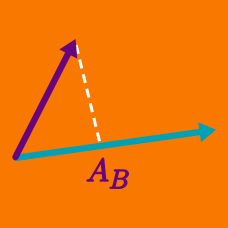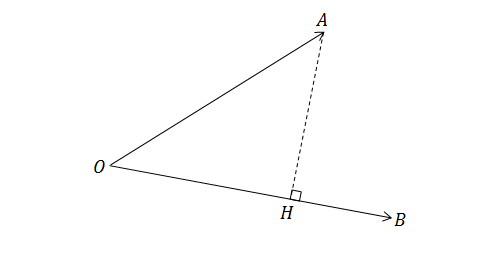Geometry

# Dot Product - Properties

If $$\vec{u} = \langle 1, 2, 3 \rangle$$ and $$\vec{v} = \langle 4, 5, 6\rangle$$, then what is $$\vec{u} \cdot \vec{v}$$?In the figure above, the point $$H$$ is the perpendicular foot from point $$A$$ on $$\overrightarrow{OB}.$$ If $$\lvert\overline{OA}\rvert=7,\lvert\overline{OB}\rvert=9,$$ and $$\lvert\overline{OH}\rvert=5,$$ what is $$\overrightarrow{OA}\cdot\overrightarrow{OB}?$$

Note: The figure is not drawn to scale.

If $$\vec{u}, \vec{v},$$ and $$\vec{w}$$ are vectors, then which of the following is not a valid operation?

\begin{align*} \text{A. } & \hspace{.35cm} (\vec{u}\cdot\vec{v}) + |\vec{w}| \\ \text{B. } & \hspace{.35cm} \vec{u}\cdot\vec{v}\cdot\vec{w} \\ \text{C. } & \hspace{.35cm} \vec{u}\cdot(\vec{v} - \vec{w}) \end{align*}

Remember! Both inputs for a dot product must be vectors.

If the vectors $$\vec{v}=(\sqrt{3},4,\sqrt{2})$$ and $$\vec{u}=(\sqrt{6},0,k)$$ are perpendicular, what must be the value of $$k?$$

Hint: If two vectors are perpendicular, then their dot product is 0.

If $$\vec{a}\cdot\vec{b}=3, \vec{a}\cdot\vec{c}=4, \vec{b}\cdot\vec{c}=-2,$$ and $$\lvert\vec{b}\rvert=1,$$ then evaluate $(\vec{a}-\vec{b})\cdot(2\vec{b}+3\vec{c})$

×

Problem Loading...

Note Loading...

Set Loading...